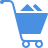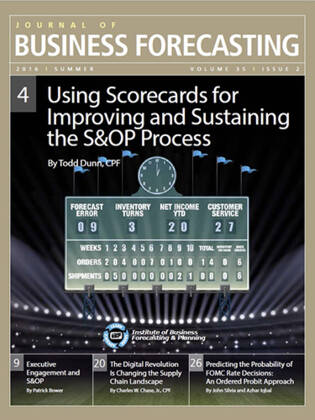Cart (0)

This article uses an ordered probit approach to estimate the six-month-out probability of three distinct scenarios of the Federal Open Market Committee (FOMC) decision: raise the fed funds target rate, reduce the rate, or keep the rate unchanged. One key finding of the ordered probit model, which may be crucial, is that since April 2013, the probability of a rate reduction has trended downward. The model has predicted an increasing probability of a rate hike since April 2013 and, at present, the probability of a rate hike is 37 percent. This pattern implies that there is a significant probability of a change in the stance of the monetary policy in the near future.

From Issue: Using Scorecards for Improving and Sustaining the S&OP Process (Summer 2016)Predicting the Probability of FOMC Rate Decisions: An Ordered Probit Approach